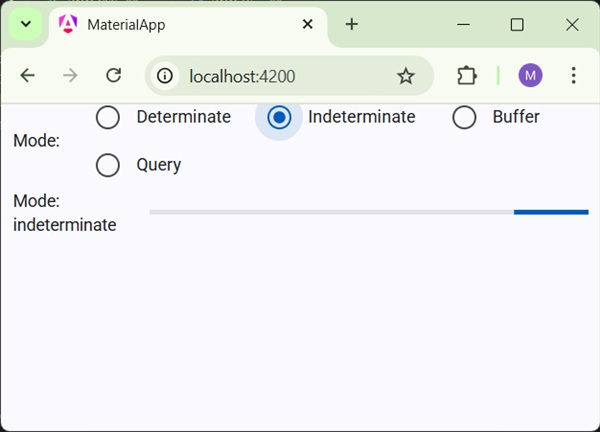# Angular Material 7 - Progress Bar

The <mat-progress-bar>, an Angular Directive, is used to show a progress bar with material styling.

In this chapter, we will showcase the configuration required to draw a deterministic as well as indeterministic progress bar using Angular Material.

## Create Angular Application

Follow the following steps to update the Angular application we created in Angular 6 - Project Setup chapter −

Step Description
1 Create a project with a name materialApp as explained in the Angular 6 - Project Setup chapter.
2 Modify app.module.ts, app.component.ts, app.component.css and app.component.html as explained below. Keep rest of the files unchanged.
3 Compile and run the application to verify the result of the implemented logic.

Following is the content of the modified module descriptor app.module.ts.

```import { BrowserModule } from '@angular/platform-browser';
import { NgModule } from '@angular/core';
import { AppComponent } from './app.component';
import {BrowserAnimationsModule} from '@angular/platform-browser/animations';
import {MatProgressBarModule, MatRadioModule, MatSliderModule} from '@angular/material'
import {FormsModule, ReactiveFormsModule} from '@angular/forms';
@NgModule({
declarations: [
AppComponent
],
imports: [
BrowserModule,
BrowserAnimationsModule,
FormsModule,
ReactiveFormsModule
],
providers: [],
bootstrap: [AppComponent]
})
export class AppModule { }
```

Following is the content of the modified ts file app.component.css.

```.tp-section {
display: flex;
align-content: center;
align-items: center;
height: 60px;
}
.tp-margin {
margin: 0 10px;
}
```

Following is the content of the modified HTML host file app.component.html.

```<section class = "tp-section">
<label class = "tp-margin">Color:</label>
<mat-radio-button class = "tp-margin" value = "primary">
Primary
<mat-radio-button class = "tp-margin" value = "accent">
Accent
<mat-radio-button class = "tp-margin" value = "warn">
Warn
</section>
<section class = "tp-section">
<label class = "tp-margin">Mode:</label>
<mat-radio-button class = "tp-margin" value = "determinate">
Determinate
<mat-radio-button class = "tp-margin" value = "indeterminate">
Indeterminate
<mat-radio-button class = "tp-margin" value = "buffer">
Buffer
<mat-radio-button class = "tp-margin" value = "query">
Query
</section>
<section class = "tp-section" *ngIf = "mode === 'determinate' || mode === 'buffer'">
<label class = "tp-margin">Progress:</label>
<mat-slider class = "tp-margin" [(ngModel)] = "value"></mat-slider>
</section>
<section class = "tp-section" *ngIf = "mode === 'buffer'">
<label class = "tp-margin">Buffer:</label>
<mat-slider class = "tp-margin" [(ngModel)] = "bufferValue"></mat-slider>
</section>
<section class = "tp-section">
<label class = "tp-margin">Mode: {{mode}}</label>
<mat-progress-bar
class = "tp-margin"
[color] = "color"
[mode] = "mode"
[value] = "value"
[bufferValue] = "bufferValue"
>
</mat-progress-bar>
</section>
```

Following is the content of the modified ts file app.component.ts.

```import { Component } from '@angular/core';
@Component({
selector: 'app-root',
templateUrl: './app.component.html',
styleUrls: ['./app.component.css']
})
export class AppComponent {
title = 'materialApp';
color = 'primary';
mode = 'determinate';
value = 50;
bufferValue = 75;
}
```

## Result

Verify the result.## Details

• Here, we've created progress bar using mat-progress-bar.